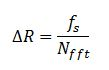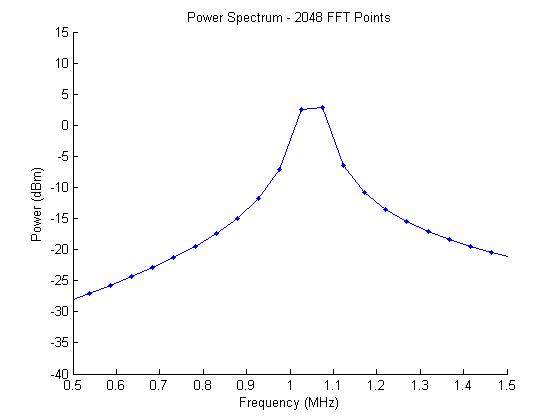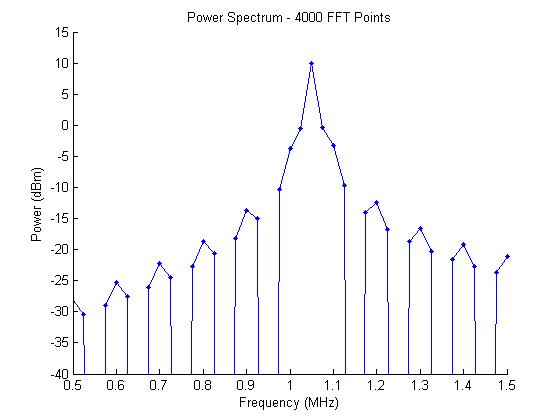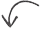# FFT Frequency Axis

Posted by in on 4-23-13

The Fast Fourier Transform (FFT) is one of the most used techniques in electrical engineering analysis, but certain aspects of the transform are not widely understood–even by engineers who think they understand the FFT.

The FFT results can be confusing for beginners, and it may not be obvious how frequency is distributed along the axis. Additionally, if you don’t pay close attention to the size of the FFT and the sampling frequency, your signal of interest may not even appear in your spectrum.

This article will show how to generate a frequency axis for the FFT in MATLAB. It will also discuss the relationship between sampling frequency and FFT size, as well as how to make sure your signals of interest are properly plotted.

## FFT Point Spacing

For the sake of this article, I’m going to assume that the time-domain signal is at least Nyquist sampled, meaning that the sampling frequency is at least twice the frequency of the maximum frequency in the time-domain signal.

The general rule of thumb is to make sure your highest frequency content in your signal of interest is no more than 40% of your sampling frequency.

The FFT shows the frequency-domain view of a time-domain signal in the frequency space of -fs/2 to fs/2, where fs is the sampling frequency. This frequency space is split into N points, where N is the number of points in the FFT. The spacing between points in frequency domain is simply expressed as:Using this equation, a frequency vector can be built in MATLAB with the following code:
f=fs/2*[-1:2/nfft:1-2/nfft];

The point +fs/2 is not part of the vector since zero frequency (DC) must be covered as well.

Choosing the Right FFT Size

Considering an example waveform with a 1 V-peak sinusoid at 1.05 MHz, let’s start exploring this concept.

Let’s start off by thinking about what we should expect to see in a power spectrum. Since the sinusoid has 1 Vpeak amplitude, we should expect to see a spike in the frequency domain with 10 dBm amplitude at 1.05 MHz.

If we use a 2048-point FFT to analyze the signal, we get the following power spectrum:Although we’ve picked a nice power of two for the FFT, the spectrum doesn’t give the expected results. The closest points in our FFT are 976.5 kHz and 1074.2 kHz, which correspond to the 10th and 11th FFT bins, respectively. That means that our true peak at 1050 kHz is not plotted!

If we change our FFT size to a number that divides 1.05 MHz more closely to an integer, we’ll see a nice peak at 10 dBm. Let’s choose 4000 points instead:Now we see a peak at the correct frequency with the correct amplitude! FFT bin 42 corresponds to the frequency 1.05 MHz.

## Why Are the Plots So Ugly?

Who wants to look at jagged plots? Not me. Seriously. If I’m going to present this sort of figure in a presentation, why not make it look a little prettier with virtually no effort?

This article uses a small number points to plot the frequency domain signals. Even though there is no true frequency resolution gained by padding the time-domain signal, you can see the FFT’s characteristic sinc function by making the size of the FFT much larger the size of your time-domain signal. Here is a plot of the same signal using 32k points.I want to stress that even though there is more fidelity to the plot, the resolution has not been improved. The space between the sinc function nulls, and thus the FFT bin size, has not increased.

If you would like a better understanding of how to increase the true resolution of the FFT, see my other article about FFT resolution and FFT zero padding.  If you’re looking for the correct way to scale the frequency domain data into a power spectrum or power spectral density in MATLAB, see those links as well.Did you enjoy this article?